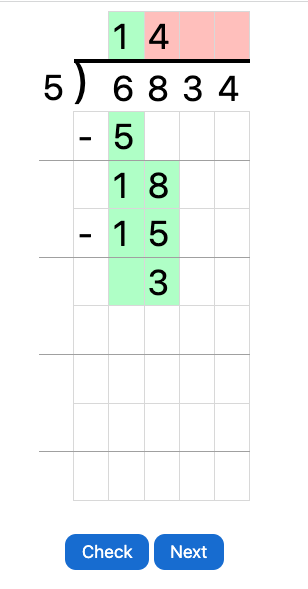MM Practice
×
Multiplication
Division
Place Value
Fractions & Decimals
Measurement
Statistics & Probability
Pre-Algebra
Money
Kindergarten
Geometry

# Long Division

## Online practice for grades 4-6

Practice long division problems step-by-step interactively and online!

Each long division problem is presented in a grid, and you can write numbers and calculate the remainders just like if you were using paper and pencil.

You can choose the number of digits for the dividend and for the divisor (actually, a range for the number of digits), to make the problems easier or more challenging.

- For fourth grade: choose single-digit divisors and 2-4 digit dividends

- For fifth grade: choose single and two-digit divisors, and 3-6 digit dividends

At any point of your practice problem, you can push the "Check" button to check your work thus far. Any wrong digits are marked red, and correct ones are marked green.

Dividend: digits
Divisor: digits
Screenshot: